## Julia Community 🟣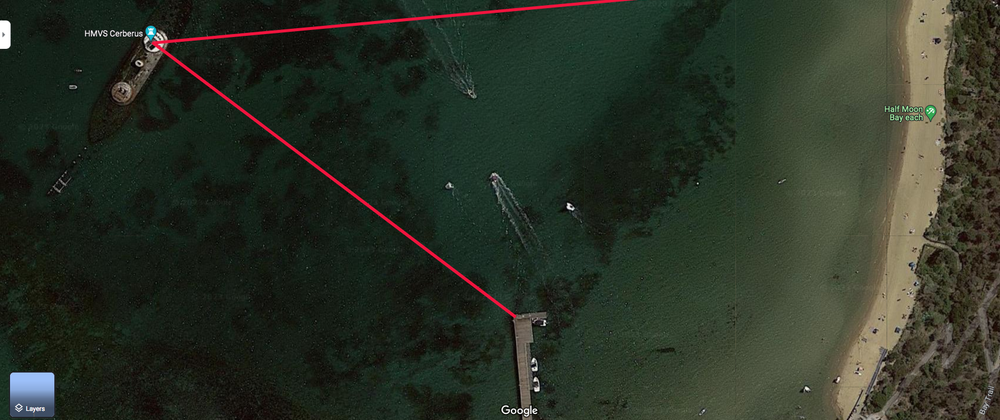Steven Siew

Posted on • Updated on

# Using Measurements.jl for Triangulation in Julia

## Finding the location of Cerberus

HMVS Cerberus is a warship that was "sunk" in blackrock. You can see the ship from shore. The objective is to find the location of HMS Cerberus without a boat.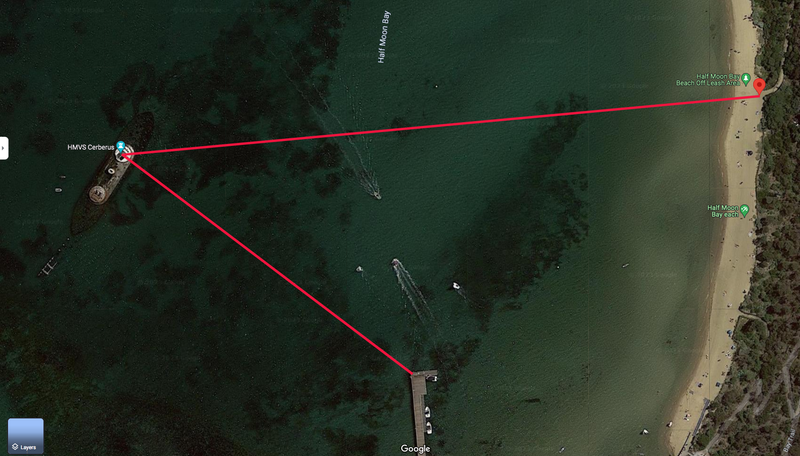You can measure the bearing to the ship from two locations

• Shore : bearing to ship is 263.3 degrees
• Pier : bearing to ship is 305.8 degrees

Using Google maps, you can get the location of the two shore locations. To get the location, right click on the spot you are interested in.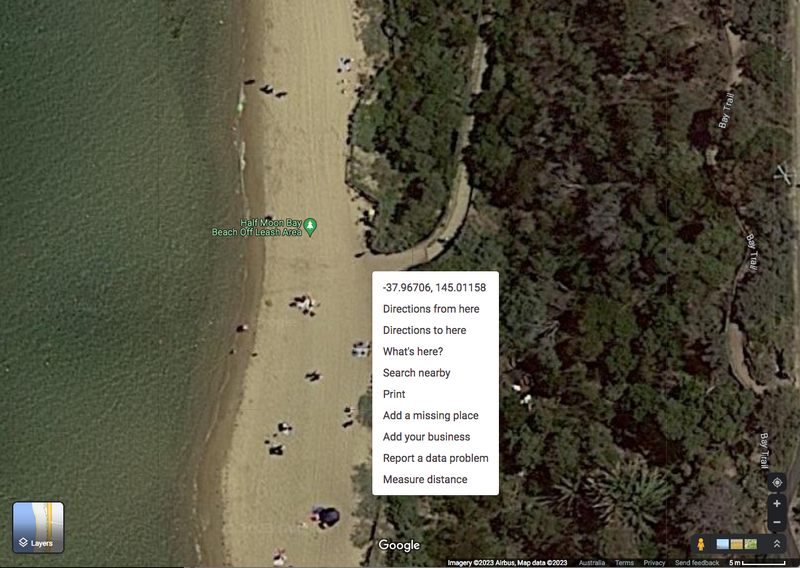• Shore : location is -37.96705678343727 (latitude), 145.01158473574677 (longtitude)
• Pier : location is -37.968305948144405 (latitude), 145.00959897290315 (longtitude)

To get the location into an XY coordinate system, we use this website and set the earth geographic datum as WGS84.

https://awsm-tools.com/lat-long-to-utm?form%5Bellipsoid%5D=WGS+84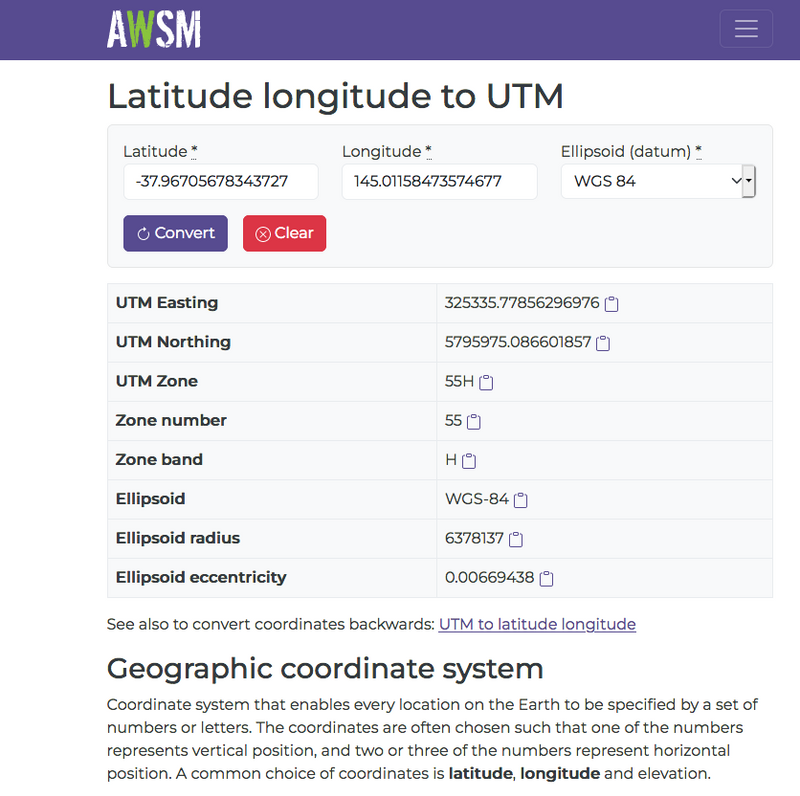This gives us the WGS84 GPS coordinates system where the numbers are in units of meters/metres. The three numbers are

• UTM ZONE (eg. 55H which is zone number 55 with Latitude Band H)
• Easting (number of meters east from a virtual base location)
• Northing (number of meters north from a virtual base location)

https://en.wikipedia.org/wiki/Universal_Transverse_Mercator_coordinate_system

Our details are

• Shore : location is 325335.77856296976 (E) 5795975.086601857 (N) 55H (UTM Zone)
• Pier : location is 325164.2938595414 (E) 5795832.741503723 (N) 55H (UTM Zone)

Now that we got all the information that we needed, we can calculate the location of the Cerebus/target

## Julia source code to calculate location

First we give you the entire source code in Julia so that you can copy and paste it into a textfile.

This source code requires the following packages

• Printf
• Measurements
using Printf

#=  Crosspoint_print(x)
Print coordinates to two decimal places
=#
function Crosspoint_print(x)
if length(x) == 1
print(rstrip(@sprintf("%-10.2f", x)))
return
end
local flag = false
print("[")
for ele in x
print(flag==true ? "," : "")
print(rstrip(@sprintf("%-10.2f", ele)))
flag = true
end
print("]")
end

Crosspoint_println(x) = begin Crosspoint_print(x); println(); end

#=  intersect2lines(A,a,B,b)

Return the intersection of two lines from
two known points A,B using sin_degree() and
cos_degree() only. a and b are the bearings
from the unknown position to the two known points A,B
=#
function intersect2lines(A,a,B,b)
den = sind(a - b)    # sind() function is Sine in degrees, cosd() is similar
tempX = B*cosd(b)*sind(a) - (A*cosd(a) + (B - A)*sind(a))*sind(b)
tempY = A*cosd(b)*sind(a) - cosd(a)*((A - B)*cosd(b) + B*sind(b))
newX = tempX/den
newY = tempY/den
return [newX,newY]
end

#=  triangulation(A,a,B,b)

Return the position of an unknown location
by triagulation from two known points A,B and
the bearing from those two known points A,B to
the unknown location
=#
triangulation(A,a,B,b) = intersect2lines(A,a+180.0,B,b+180.0)

Pos_Shore = (325335.78,5795975.09)    # Easting 325335.78 Northing 5795975.09
Pos_Shore_bearing_to_target = 263.3   # 263.3 degrees

Pos_Pier  = (325164.29,5795832.74)    # Easting 325164.29 Northing 5795832.74
Pos_Pier_bearing_to_target  = 305.8   # 305.8 degrees

println("program start")

println("The coordinates for :")
print("Shore is ")
Crosspoint_println(Pos_Shore)
print("Pier is ")
Crosspoint_println(Pos_Pier)
println("")
println("Bearing from shore to cerberus/target is ",round(Pos_Shore_bearing_to_target,digits=1))
println("Bearing from pier to cerberus/target is ",round(Pos_Pier_bearing_to_target,digits=1))
println("")

target_pos_estimate = triangulation(Pos_Shore,
Pos_Shore_bearing_to_target,
Pos_Pier,
Pos_Pier_bearing_to_target)

print("The estimated position of target is ")
Crosspoint_println(target_pos_estimate)
println("In coordinate format of [Easting value,Northing value]")

println("")
println("Now we do the same thing again using measurement")
println("")

#
# Now use Measurement to measure the uncertainties
#    help?>  ±
#    "±" can be typed by \pm<tab>

using Measurements

Shore = (325335.78 ± 5.0,5795975.09 ± 5.0)
Pier  = (325164.29 ± 5.0,5795832.74 ± 5.0)
Shore_bearing_to_target = 263.3 ± 0.5
Pier_bearing_to_target  = 305.8 ± 0.5

target_pos_estimate2 = triangulation(Shore,
Shore_bearing_to_target,
Pier,
Pier_bearing_to_target)

value_two_decimal_places(m)       = rstrip(  @sprintf("%-16.2f",Measurements.value(m))  )
uncertainty_two_decimal_places(m) = rstrip(  @sprintf("%-16.2f",Measurements.uncertainty(m))  )

print("The estimated position of target using Measurements package is ")
print("[")
print(value_two_decimal_places(target_pos_estimate2))
print(" ± ")
print(uncertainty_two_decimal_places(target_pos_estimate2))
print(",")
print(value_two_decimal_places(target_pos_estimate2))
print(" ± ")
print(uncertainty_two_decimal_places(target_pos_estimate2))
println("]")
println("")

dict_uncertainty_in_easting  = Measurements.uncertainty_components(target_pos_estimate2)
dict_uncertainty_in_northing = Measurements.uncertainty_components(target_pos_estimate2)

println("Uncertainty in the easting value")
for key in keys(dict_uncertainty_in_easting)
println(key," => ",dict_uncertainty_in_easting[key])
end
println("")

println("Uncertainty in the northing value")
for key in keys(dict_uncertainty_in_northing)
println(key," => ",dict_uncertainty_in_northing[key])
end


Now we display the screen output of the program

program start
The coordinates for :
Shore is [325335.78,5795975.09]
Pier is [325164.29,5795832.74]

Bearing from shore to cerebus/target is 263.3
Bearing from pier to cerebus/target is 305.8

The estimated position of target is [325018.58,5795937.83]
In coordinate format of [Easting value,Northing value]

Now we do the same thing again using measurement

The estimated position of target using Measurements
package is [325018.58 ± 10.32,5795937.83 ± 5.04]

Uncertainty in the easting value
(5.79583274e6, 5.0, 0x0000000000000004) => 5.961637475221173
(263.3, 0.5, 0x0000000000000005) => 3.345995971168122
(305.8, 0.5, 0x0000000000000006) => 2.304707563592274
(5.79597509e6, 5.0, 0x0000000000000002) => 5.961637475221173
(325164.29, 5.0, 0x0000000000000003) => 4.299668552553106
(325335.78, 5.0, 0x0000000000000001) => 0.7003314474468948

Uncertainty in the northing value
(5.79583274e6, 5.0, 0x0000000000000004) => 0.7003314474468948
(263.3, 0.5, 0x0000000000000005) => 2.4132084035663866
(5.79597509e6, 5.0, 0x0000000000000002) => 4.299668552553106
(305.8, 0.5, 0x0000000000000006) => 0.27074091483518714
(325335.78, 5.0, 0x0000000000000001) => 0.5050949698748158
(325164.29, 5.0, 0x0000000000000003) => 0.5050949698748158
julia>


Next we will talk about each section of the Julia program to explain how it is accomplished.

### Crosspoint_print()

Now we will talk about the function Crosspoint_print() and Crosspoingg_println()

using Printf

#=  Crosspoint_print(x)
Print coordinates to two decimal places
=#
function Crosspoint_print(x)
if length(x) == 1
print(rstrip(@sprintf("%-10.2f", x)))
return
end
local flag = false
print("[")
for ele in x
print(flag==true ? "," : "")
print(rstrip(@sprintf("%-10.2f", ele)))
flag = true
end
print("]")
end

Crosspoint_println(x) = begin Crosspoint_print(x); println(); end


Crosspoint_print() will printout a floating point number (or a tuple or an array of floating point number) to two decimal places.

For example:

julia> Crosspoint_print(pi)
3.14
julia> Crosspoint_print([pi,2*pi])
[3.14,6.28]
julia> Crosspoint_print([pi,2*pi,pi^2])
[3.14,6.28,9.87]
julia> Crosspoint_print((pi,2*pi,pi^2))
[3.14,6.28,9.87]


The purposes of Crosspoint_print() is to easily print out the coordinates. For example:

julia> Pos_Shore = (325335.78,5795975.09)
(325335.78, 5.79597509e6)

julia> print(  Pos_Shore  )
(325335.78, 5.79597509e6)

julia> Crosspoint_print(  Pos_Shore  )
[325335.78,5795975.09]


### intersect2lines() and triangulation()

Now we will talk about the function intersect2lines() and triangulation()

#=  intersect2lines(A,a,B,b)

Return the intersection of two lines from
two known points A,B using sin_degree() and
cos_degree() only. a and b are the bearings
from the unknown position to the two known points A,B
=#
function intersect2lines(A,a,B,b)
den = sind(a - b)    # sind() function is Sine in degrees, cosd() is similar
tempX = B*cosd(b)*sind(a) - (A*cosd(a) + (B - A)*sind(a))*sind(b)
tempY = A*cosd(b)*sind(a) - cosd(a)*((A - B)*cosd(b) + B*sind(b))
newX = tempX/den
newY = tempY/den
return [newX,newY]
end

#=  triangulation(A,a,B,b)

Return the position of an unknown location
by triagulation from two known points A,B and
the bearing from those two known points A,B to
the unknown location
=#
triangulation(A,a,B,b) = intersect2lines(A,a+180.0,B,b+180.0)


In trigonometry and geometry, triangulation is the process of determining the location of a point by forming triangles to the point from two (or more) known points.

So the question is "How do we develop triangulation?"

We start from FIRST PRINCIPLES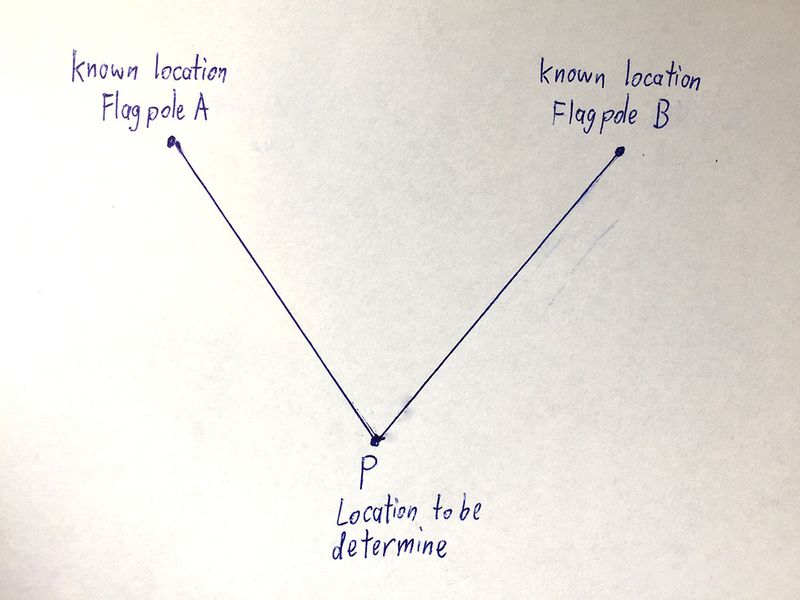Next we assume we knew the equation of the line (aka Y = M * X + C ) and work out the location of the unknown location.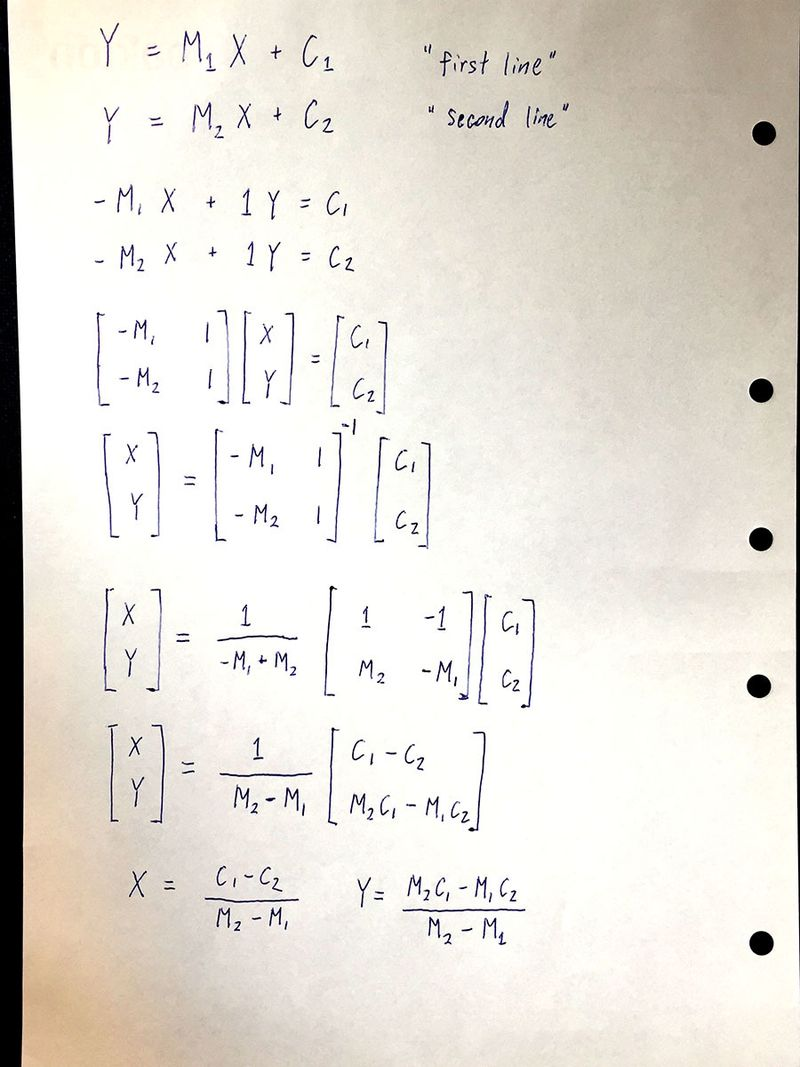Now to find the value of X and Y, both X & Y at point P , all we have to do is to find the values of

• M1
• C1
• M2
• C2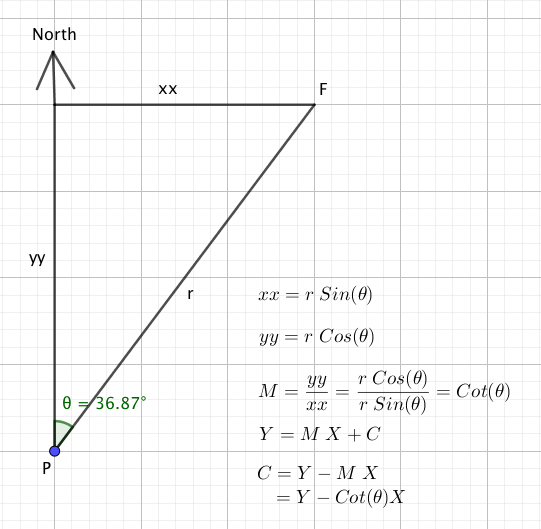So now we know that

• M1 = Cot(bearing to flagpole A)
• M2 = Cot(bearing to flagpole B)
• C1 = Y_flagpoleA - Cot(bearing to flagpole A) * X_flagpoleA
• C2 = Y_flagpoleB - Cot(bearing to flagpole B) * X_flagpoleB

Next we put together everything that we know

The value for X is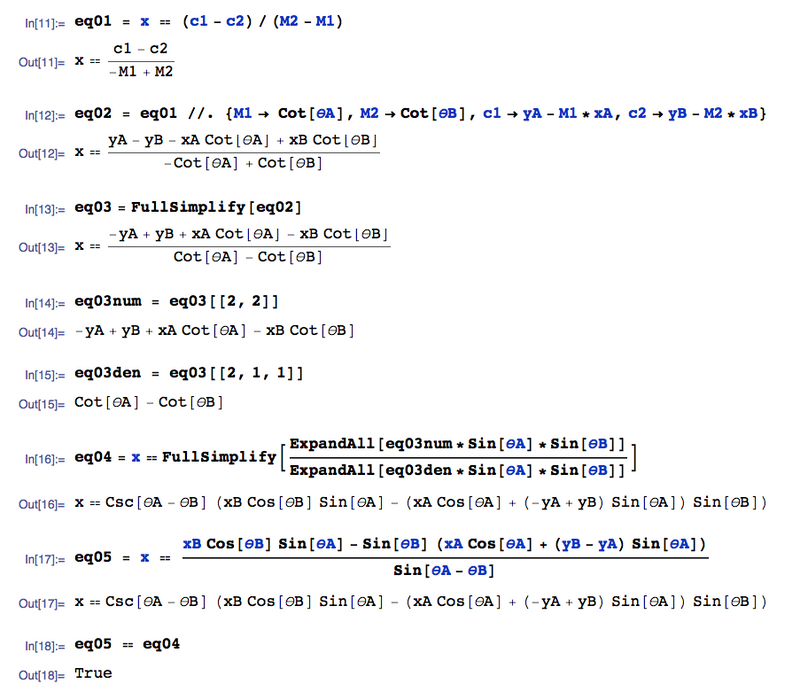The value for Y is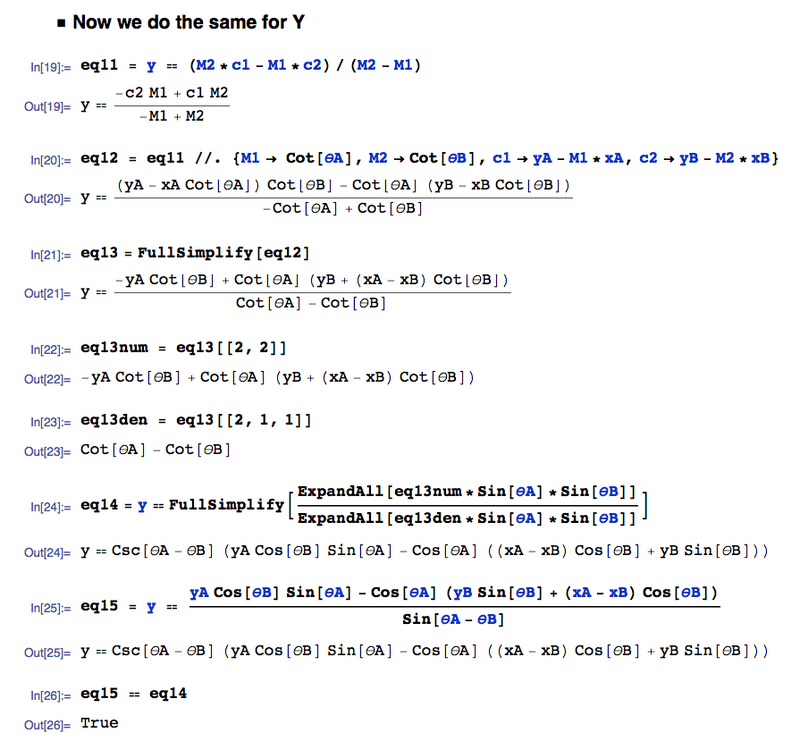And so in SUMMARY, the location of unknown location is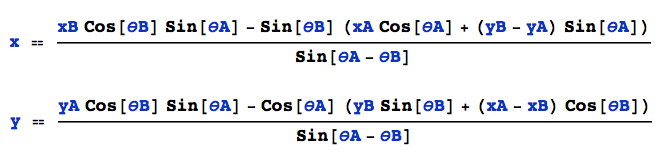Which is what is in our function intersect2lines(A,a,B,b)

function intersect2lines(A,a,B,b)
den = sind(a - b)    # sind() function is Sine in degrees, cosd() is similar
tempX = B*cosd(b)*sind(a) - (A*cosd(a) + (B - A)*sind(a))*sind(b)
tempY = A*cosd(b)*sind(a) - cosd(a)*((A - B)*cosd(b) + B*sind(b))
newX = tempX/den
newY = tempY/den
return [newX,newY]
end


Regarding the function triangulation()

The difference between intersect2lines() and triangulation() is very simple.

The function intersect2lines() assumes that you are in location P and you have the following two bearings:

• bearing a from location P to Flagpole A
• bearing b from location P to Flagpole B

Whereas the function triangulation() assumes that you are
at the two Flagpoles and you have the following two bearings:

• bearing a from Flagpole A to location P
• bearing b from Flagpole B to location P

So you see the only difference is 180 degrees so

triangulation(A,a,B,b) = intersect2lines(A,a+180.0,B,b+180.0)


### Location of Cerberus

So with the Julia code

target_pos_estimate = triangulation(Pos_Shore,
Pos_Shore_bearing_to_target,
Pos_Pier,
Pos_Pier_bearing_to_target)

print("The estimated position of target is ")
Crosspoint_println(target_pos_estimate)
println("In coordinate format of [Easting value,Northing value]")


We can now get the location of the Cerberus

The estimated position of target is [325018.58,5795937.83]
In coordinate format of [Easting value,Northing value]


Once you got the location of Cerberus in UTM format, you can convert it back to Latitude and Longitude using this website

https://awsm-tools.com/utm-to-lat-long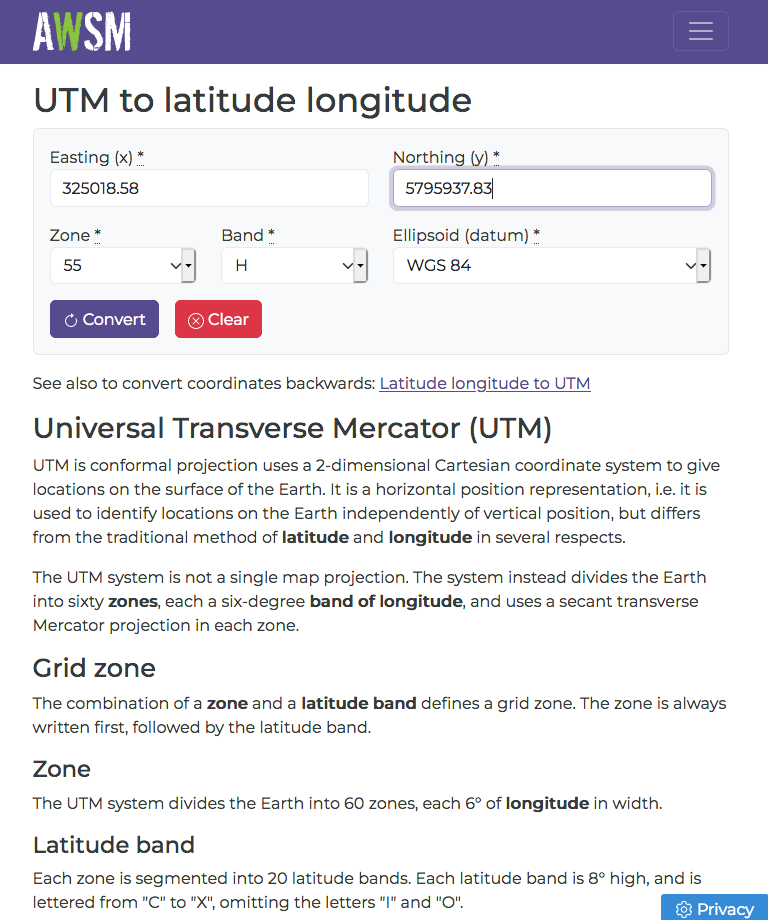### Now we do the same thing again but this time with Measurements

Measurements is a Julia package that allows us to include (the amount of) uncertainties in our input values.

To input uncertainties, we type '\' , 'p' , 'm' , <TAB> on the REPL or a Julia Editor.

#
# Now use Measurement to measure the uncertainties
#    help?>  ±
#    "±" can be typed by \pm<tab>

using Measurements

Shore = (325335.78 ± 5.0,5795975.09 ± 5.0)
Pier  = (325164.29 ± 5.0,5795832.74 ± 5.0)
Shore_bearing_to_target = 263.3 ± 0.5
Pier_bearing_to_target  = 305.8 ± 0.5


For example: Shore_bearing_to_target = 263.3 ± 0.5

The variable "Shore_bearing_to_target" is a measurement.
The measurement "Shore_bearing_to_target" has two components.

• The first component is called "value"
• The second component is called "uncertainty"

This function returns the "value" of a measurement

• Measurements.value()

This other function returns the "uncertainty" of a measurement

• Measurements.uncertainty()

I have the following two functions to return the numeric value of the two above components in a string

value_two_decimal_places(m)       = rstrip(  @sprintf("%-16.2f",Measurements.value(m))  )
uncertainty_two_decimal_places(m) = rstrip(  @sprintf("%-16.2f",Measurements.uncertainty(m))  )


### The location with uncertainties

So here is the location with uncertainties

target_pos_estimate2 = triangulation(Shore,
Shore_bearing_to_target,
Pier,
Pier_bearing_to_target)

print("The estimated position of target using Measurements package is ")
print("[")
print(value_two_decimal_places(target_pos_estimate2))
print(" ± ")
print(uncertainty_two_decimal_places(target_pos_estimate2))
print(",")
print(value_two_decimal_places(target_pos_estimate2))
print(" ± ")
print(uncertainty_two_decimal_places(target_pos_estimate2))
println("]")
println("")


Here is the output

Now we do the same thing again using measurement

The estimated position of target using Measurements
package is [325018.58 ± 10.32,5795937.83 ± 5.04]


### Relative contributions to the uncertainties

dict_uncertainty_in_easting  = Measurements.uncertainty_components(target_pos_estimate2)
dict_uncertainty_in_northing = Measurements.uncertainty_components(target_pos_estimate2)

println("Uncertainty in the easting value")
for key in keys(dict_uncertainty_in_easting)
println(key," => ",dict_uncertainty_in_easting[key])
end
println("")

println("Uncertainty in the northing value")
for key in keys(dict_uncertainty_in_northing)
println(key," => ",dict_uncertainty_in_northing[key])
end


Consider the uncertainties for the "northing" of Cerberus

5795937.83 ± 5.04

The uncertainty for the northing is 5.04 but where does it comes from?

It came from the following input measurements

• Shore Easting
• Shore Northing
• Shore_bearing_to_target
• Pier Easting
• Pier Northing
• Pier_bearing_to_target

There is a way to determine the "relative" contribution to the final uncertainty.

Uncertainty in the northing value
(5.79583274e6, 5.0, 0x0000000000000004) => 0.7003314474468948
(263.3, 0.5, 0x0000000000000005) => 2.4132084035663866
(5.79597509e6, 5.0, 0x0000000000000002) => 4.299668552553106
(305.8, 0.5, 0x0000000000000006) => 0.27074091483518714
(325335.78, 5.0, 0x0000000000000001) => 0.5050949698748158
(325164.29, 5.0, 0x0000000000000003) => 0.5050949698748158


Here we see that the value 5795975.09 (Which is Shore Northing) contributes 4.29966 of relative weight to the final uncertainty of the northing value of Cerberus and this INPUT parameter is THE BIGGEST contribution.

This means that if you reduce the uncertainty for Shore Northing, you will get the biggest bang per buck for lowering the final uncertainty for "northing" of the Cerberus.

This mean that if you want to get the biggest bang per buck for lowering the final uncertainty for "northing" of the Cerberus, you should put all your effort into reducing the uncertainty for Shore Northing.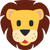With a compass. You stand at the location, look towards the Target and then read the direction on the compass. The direction on your compass is the bearing from your current location to the Target. Watch the Youtube Video youtu.be/-Ak86suJFjo

postscript: do not forget to correct for magnetic declination

Melbourne Victoria
Latitude: 37° 48' 50.4" S
Longitude: 144° 57' 48" E
MELBOURNE
Magnetic Declination: +11° 50'
Declination is POSITIVE (EAST)
Inclination: 68° 46'
Magnetic field strength: 60012.2 nT